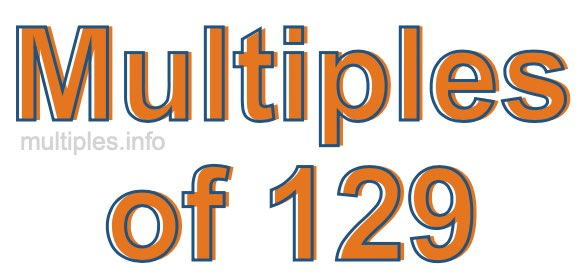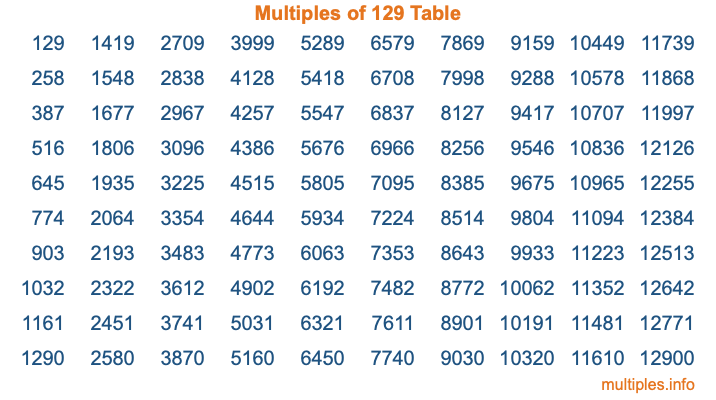Multiples of 129Welcome to the Multiples of 129 page. Here we will first teach you everything you will ever need to know about the multiples of 129, and then give you a study guide summary of everything we taught you to make sure you remember it all. Use this page to look up facts and learn information about the multiples of 129. This page will make you a multiples of one hundred twenty-nine expert!

Definition of Multiples of 129
Multiples of 129 are all the numbers that when divided by 129 equal an integer. Each of the multiples of 129 are called a multiple. A multiple of 129 is created by multiplying 129 by an integer.

Therefore, to create a list of multiples of 129, you start with 1 multiplied by 129, then 2 multiplied by 129, then 3 multiplied by 129, and so on for as long as you want. Thus, the list of the first five multiples of 129 is 129, 258, 387, 516, and 645. To see a larger list of multiples of 129, see the printable image of Multiples of 129 further down on this page. We also have a category where you can choose any nth multiple of 129.

Multiples of 129 Checker
The Multiples of 129 Checker below checks to see if any number of your choice is a multiple of 129. In other words, it checks to see if there is any number (integer) that when multiplied by 129 will equal your number. To do that, we divide your number by 129. If the the quotient is an integer, then your number is a multiple of 129.

Is  a multiple of 129?

Least Common Multiple of 129 and ...
A Least Common Multiple (LCM) is the lowest multiple that two or more numbers have in common. This is also called the smallest common multiple or lowest common multiple and is useful to know when you are adding our subtracting fractions. Enter one or more numbers below (129 is already entered) to find the LCM.

Check out our LCM Calculator if you need more details about the Least Common Multiple or if you need the LCM for different numbers for adding and subtraction fractions.

nth Multiple of 129
As we stated above, 129 is the first multiple of 129, 258 is the second multiple of 129, 387 is the third multiple of 129, and so on. Enter a number below to find the nth multiple of 129.

th multiple of 129

Multiples of 129 vs Factors of 129
129 is a multiple of 129 and a factor of 129, but that is where the similarities end. All postive multiples of 129 are 129 or greater than 129. All positive factors of 129 are 129 or less than 129.

Below is the beginning list of multiples of 129 and the factors of 129 so you can compare:

Multiples of 129: 129, 258, 387, 516, 645, etc.

Factors of 129: 1, 3, 43, 129

As you can see, the multiples of 129 are all the numbers that you can divide by 129 to get a whole number. The factors of 129, on the other hand, are all the whole numbers that you can multiply by another whole number to get 129.

It's also interesting to note that if a number (x) is a factor of 129, then 129 will also be a multiple of that number (x).

Multiples of 129 vs Divisors of 129
The divisors of 129 are all the integers that 129 can be divided by evenly. Below is a list of the divisors of 129.

Divisors of 129: 1, 3, 43, 129

The interesting thing to note here is that if you take any multiple of 129 and divide it by a divisor of 129, you will see that the quotient is an integer.

Multiples of 129 Table
Below is an image of the first 100 multiples of 129 in a table. The table is in chronological order, column by column. The first column has the first ten multiples of 129, the second column has the next ten multiples of 129, and so on.The Multiples of 129 Table is also referred to as the 129 Times Table or Times Table of 129. You are welcome to print out our table for your studies.

Negative Multiples of 129
Although not often discussed or needed in math, it is worth mentioning that you can make a list of negative multiples of 129 by multiplying 129 by -1, then by -2, then by -3, and so on, to get the following list of negative multiples of 129:

-129, -258, -387, -516, -645, etc.

Multiples of 129 Summary
Below is a summary of important Multiples of 129 facts that we have discussed on this page. To retain the knowledge on this page, we recommend that you read through the summary and explain to yourself or a study partner why they hold true.

There are an infinite number of multiples of 129.

A multiple of 129 divided by 129 will equal a whole number.

129 divided by a factor of 129 equals a divisor of 129.

The nth multiple of 129 is n times 129.

The largest factor of 129 is equal to the first positive multiple of 129.

129 is a multiple of every factor of 129.

129 is a multiple of 129.

A multiple of 129 divided by a divisor of 129 equals an integer.

129 divided by a divisor of 129 equals a factor of 129.

Any integer times 129 will equal a multiple of 129.

Multiples of a Number
Here you can get the multiples of another number, all with the same attention to detail as we did for multiples of 129 on this page.

Multiples of
Multiples of 130
Did you find our page about multiples of one hundred twenty-nine educational? Do you want more knowledge? Check out the multiples of the next number on our list!